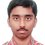Number Theory Problem

Show that $19^{93}$ - $13^{99}$ is a positive integer divisible by 162.

NOTE:Actually I could not understand how to proceed. Can anyone show me the path ?Note by Nishant Sharma
6 years, 4 months ago

This discussion board is a place to discuss our Daily Challenges and the math and science related to those challenges. Explanations are more than just a solution — they should explain the steps and thinking strategies that you used to obtain the solution. Comments should further the discussion of math and science.

When posting on Brilliant:

• Use the emojis to react to an explanation, whether you're congratulating a job well done , or just really confused .
• Ask specific questions about the challenge or the steps in somebody's explanation. Well-posed questions can add a lot to the discussion, but posting "I don't understand!" doesn't help anyone.
• Try to contribute something new to the discussion, whether it is an extension, generalization or other idea related to the challenge.
• Stay on topic — we're all here to learn more about math and science, not to hear about your favorite get-rich-quick scheme or current world events.

MarkdownAppears as
*italics* or _italics_ italics
**bold** or __bold__ bold

- bulleted
- list

• bulleted
• list

1. numbered
2. list

1. numbered
2. list
Note: you must add a full line of space before and after lists for them to show up correctly
paragraph 1

paragraph 2

paragraph 1

paragraph 2

> This is a quote
This is a quote
# I indented these lines
# 4 spaces, and now they show
# up as a code block.

print "hello world"
# I indented these lines
# 4 spaces, and now they show
# up as a code block.

print "hello world"
MathAppears as
Remember to wrap math in $$...$$ or $...$ to ensure proper formatting.
2 \times 3 $2 \times 3$
2^{34} $2^{34}$
a_{i-1} $a_{i-1}$
\frac{2}{3} $\frac{2}{3}$
\sqrt{2} $\sqrt{2}$
\sum_{i=1}^3 $\sum_{i=1}^3$
\sin \theta $\sin \theta$
\boxed{123} $\boxed{123}$

Sort by:

What I had done is that I wrote it as $(18+1)^{93}-(12+1)^{99}$ and now it is easy to see that a very few terms are left, after the binomial expansion, that are not divisible by $162$,i.e., $3^4*2$. And then it is very easy to show that the remaining terms add up to be a multiple of $162$ as well.

- 6 years, 4 months ago

latex not loading proprly pls type it

- 6 years, 4 months ago

Another method is to note that $13^2 \equiv 7 \pmod {162}$.

- 6 years, 4 months ago

We will have to prove that its divisible by $81$ and $2$. But its obviously divisible by $2$ and if we consider both numbers mod 9, it turns out that its divisible by $9$.

- 6 years, 4 months ago

Got that it is divisible by 2 and 9. But we need to prove that it is divisible by 162. Anyways, I could not think of approaching it this way.

- 6 years, 4 months ago

it goes for 81 also

- 6 years, 4 months ago

hey vikram bhaiya please mujhe yei problem latex chor kar likh do mere computer par load nehi ho raha latex

- 6 years, 4 months ago

show that 19^93 - 13^99 is a positive integer divisible by 162

- 6 years, 4 months ago

thanks

- 6 years, 4 months ago

right but first prove 19^93>13^99

- 6 years, 4 months ago

not needed to show the greater than sign.

- 6 years, 4 months ago

yes if we do bionomial then not but otherwise Show that 19^93 - 13^99 is a positive integer

- 6 years, 4 months ago

inequality korchhis bole sob jaegae inequality? positivity or negativity is not at all needed as the problem is asking to prove divisibility.

- 6 years, 4 months ago

hmm thikie bolecho go sohomda

- 6 years, 4 months ago

But why is it required?

- 6 years, 4 months ago

yeah i got my mistake

- 6 years, 4 months ago

A way to reduce the bulk of this problem is to use Euler's totient function. Also, it is inevitably evident that the given number is divisible by $2$, so we have to worry about $81$ only.

After applying Euler's totient function, we only need to show that: $19^{39} \equiv c \pmod{81}$ and $13^{45} \equiv c \pmod{81}$ i.e $19^{39}$ and $13^{45}$ leave the same remainder namely $"c"$ on division by $81$.

- 6 years, 4 months ago